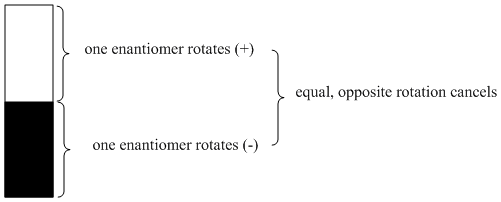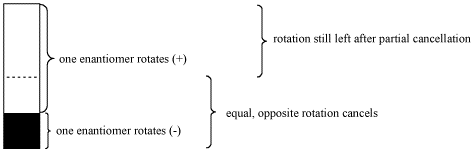Optical Rotation

• An equal mixture of two enantiomers is called a racemic mixture or racemate.
• If two enantiomers rotate plane-polarized light in opposite directions, a racemate will not rotate light at all. The effects of the two enantiomers will cancel out.Figure SC6.1. Optical rotation canceled out in a racemic mixture.Figure SC6.2. Optical rotation only partially canceled in a non-racemic mixture of enantiomers.

• If two enantiomers are present in an unequal ratio, only part of the optical rotation will be canceled out.
• By comparing the rotation of the sample to the rotation of a pure enantiomer, the enantiomeric purity can be determined.

The "optical purity" is a comparison of the optical rotation of a pure sample of unknown stereochemistry versus the optical rotation of a sample of pure enantiomer. It is expressed as a percentage. If the sample only rotates plane-polarized light half as much as expected, the optical purity is 50%.

Optical purity also corresponds to "enantiomeric excess". If the unknown sample rotates light 50% as much as a sample of pure enantiomer, it must contain 50% enantiomeric excess; the other 50% is a racemic mixture. In other words, if the sample is 75% of one enantiomer and 25% of the other, 50% of the mixture will simply cancel out in terms of optical activity. The remaining 50% will still exert optical activity, but only half as much as if the sample were 100% of that enantiomer.

These relationships could be expressed in formulae:

Optical purity (op) = [ (optical rotation of pure compound) / (optical rotation of pure enantiomer) ] x 100%

Enantiomeric excess (ee) = optical purity (that is, these numbers are always the same, although they represent different things)

% major enantiomer = enantiomeric excess + [ (100 - enantiomeric excess) / 2 ] = 50 + (enantiomeric excess / 2)

% minor enantiomer = 100 - % major enantiomer

Problem SC6.1.

The (+) enantiomer of compound A has an optical rotation of 75o. If a pure sample of compound A has an optical rotation of 50o, what is the composition of the sample?

Problem SC6.2.

The (+) enantiomer of compound B has an optical rotation of 50o. If a pure sample of B contains 10% of the (+) enantiomer and 90% of the (-) enantiomer, what is the optical rotation value?